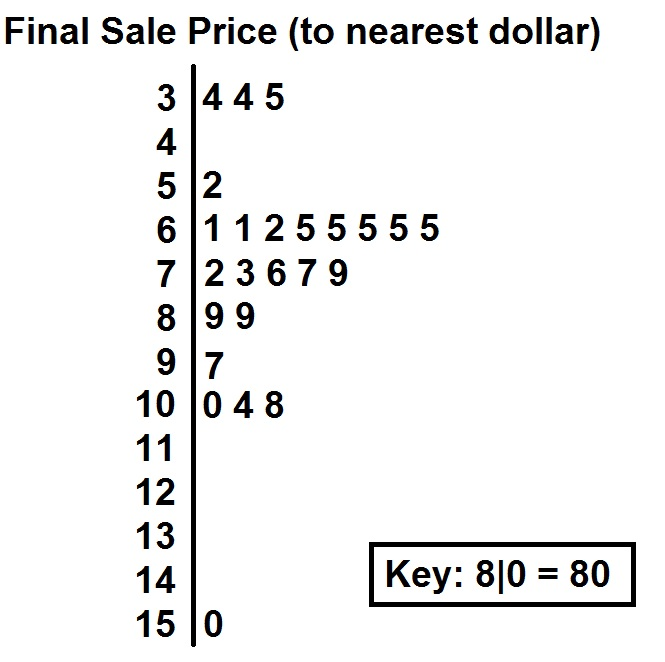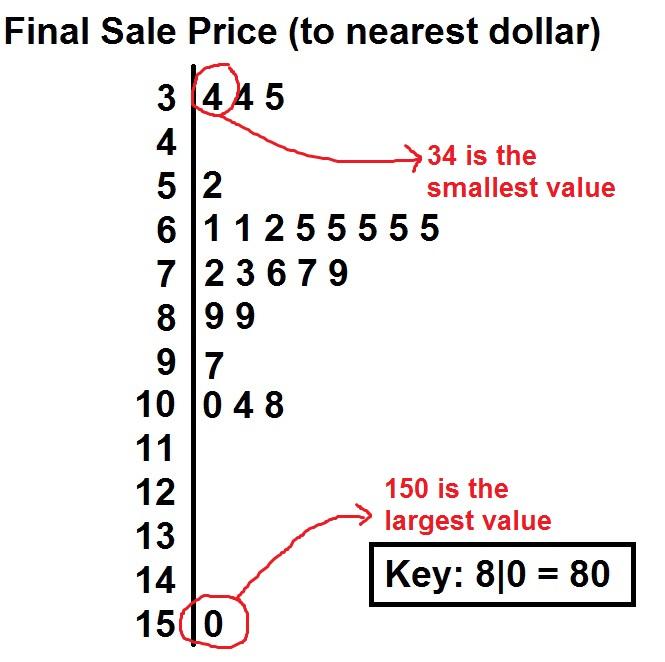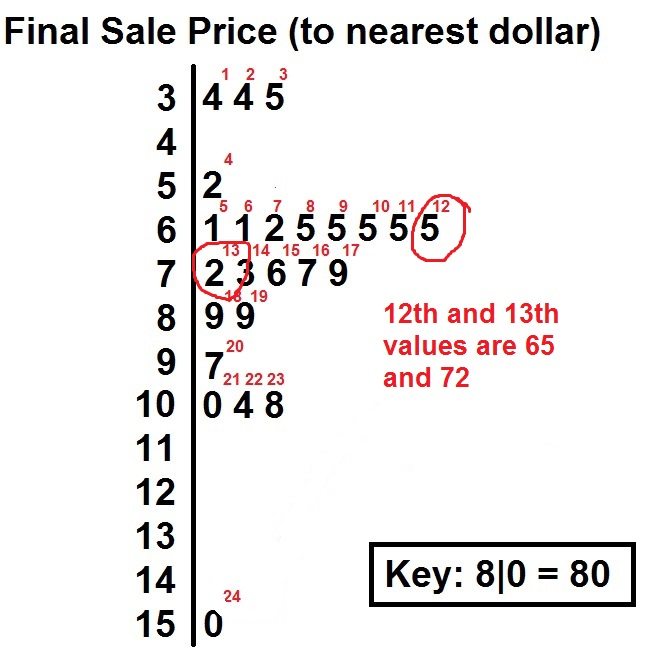# How to read a stemplot

Stemplots are sometimes called stem and leaf plots because each number in the original data set has been broken up into two pieces: a stem and a leaf. When we read stemplots, we need to keep this in mind. For any stemplot, you will see the leaves on the right hand side of the bar. Each leaf represents a single value in the data set. Using this idea, let’s try to answer some questions.

## Answering questions with a stemplot

So let’s suppose that someone has been selling their old baseball card collection online card by card. They have kept track of all of their sales so far and plotted them on the stemplot below.Using this, we will answer the following questions about the sale prices so far.

(a) How many cards have they sold?
(b) What was the lowest sale price? the highest?
(c) What is the median sale price?
(d) What is the mode?

From the key, we can see that the leafs represent the ones digit. This is important to consider when answering these questions!

### (a) How many cards have they sold?

Since each leaf represents a single data value, we can just count the number of leaves in the stemplot.Based on this, so far they have sold 24 total cards.

### (b) What is the lowest sale price? the highest?

The values on the stemplot are all in order from smallest to largest (if you look from top to bottom).The lowest sale price of any card so far is $34 while the highest is$150.

### (c) What is the median sale price?

Recall that the median is the middle value, when the values are placed in order from smallest to largest. If there are an even number of values, then it is the average of the middle two. In this data set, there are 24 values. The middle two values would be the 12th and 13th values.The median sale price is:

$$\dfrac{65 + 72}{2} = \68.50$$